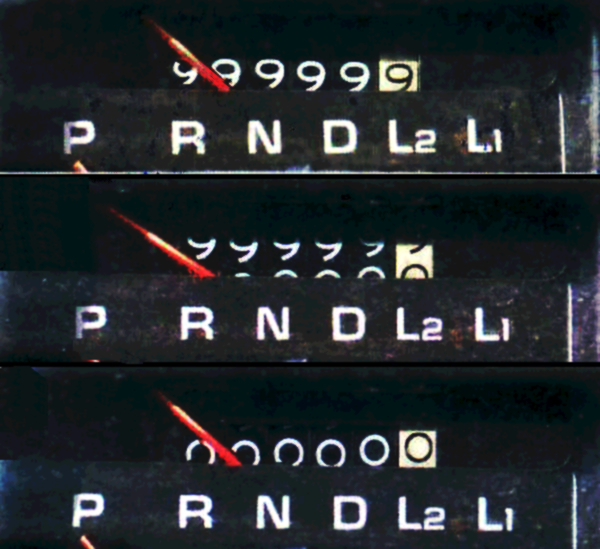Yes, the formula above is correct. Well, it depends on what we mean by correct.

## NDVI does not make sense#

Imagine the following situation. We have fetched a cloud-free mosaic of Sentinel 2 satellite data and want to measure NDVI (Normalised difference vegetation index), which uses red and near-infrared bands within this simple formula.

NDVI = (NIR - Red) / (NIR + Red)

The results are normalised, which in this case means that they lie between -1 and 1. Always.

We open out raster data using `xarray` and have all 4 bands in a single `xarray.DataSet`. The code to measure NDVI is then simple.

``````>>> red = data.sel(band=1)  # select red band
>>> nir = data.sel(band=4)  # select near-infrared band

>>> ndvi = (nir - red) / (nir + red)  # compute NDVI
``````

And, a surprise! Our results are between 0 and 170. That is certainly not correct. What has happened?

## 16-bit unsigned integer#

The data coming from Sentinel 2 are stored as 16-bit unsigned integer (`uint16`). That means that the value the array can hold can be anything between 0 and 216 - 1 (65,535). Remember that NDVI is between -1 and 1. Does it mean that `uint16` cannot represent NDVI values? Yes, precisely.

Look at this toy example to understand what is happening during the computation of NDVI in `uint16`. Let’s have an array with four numbers and subtract 10 from each of them.

``````>>> array = numpy.array([1, 3, 6, 9], dtype='uint16')
>>> array - 10

array([65527, 65529, 65532, 65535], dtype=uint16)
``````

Yes, as weird as it is, it is correct. The result should be negative, but we can’t have negative values in `uint16`. So what happens is that the counter rolls over and subtracts the remaining value from the maximum it can represent (65,535 - x).

It is exactly like a rollover of the odometer. We ran out of values, so they started over. The only difference is that we have 16 binary values encoding each number, not decimal.The fix is easy. We have to use data type which does not limit you like this, like a 64-bit integer.

``````>>> array.astype('int64') - 10

array([-9, -7, -4, -1])
``````

Compared to a 64-bit integer, a 16-bit integer is efficient since the resulting file will be much smaller (that is why it is used in the first place) but it can be limiting.

Be aware of your data types, so you don’t make the same mistake we did ;).